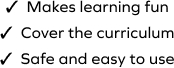# Estimate the Sum

SplashLearn - The complete PreK - Grade 5 Math & ELA Learning Program Built for Your Child
Home > Estimate the Sum
The game requires students to recall concepts related to rounding decimal numbers and estimate the answer to move towards mastery in the topic. Your young mathematician will learn to estimate the sum of two decimal numbers in this game. Students will need to analyze and select the correct answer from a set of given options.